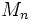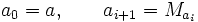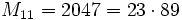# Infinitude of Poulet numbers

## Statement

There exist infinitely many Poulet numbers, i.e., there infinitely many odd composite numberssuch that:.

## Proof

By fact (1), ifis a Poulet number, the Mersenne numberis either prime or a Poulet number. But by fact (2), it cannot be prime. Thus, the Mersenne number for a Poulet number is a Poulet number.

Thus, supposeis a Poulet number. Consider the sequence:.

In other words, each member of the sequence is the Mersenne number for the preceding number. This is a strictly increasing sequence, and each member of it is a Poulet number, so if there is one Poulet number, there are infinitely many Poulet numbers.

Thus, it is sufficient to find just one Poulet number. Using fact (1), consider. This is not prime, so by fact (1), it is a Poulet number, and this completes the proof.